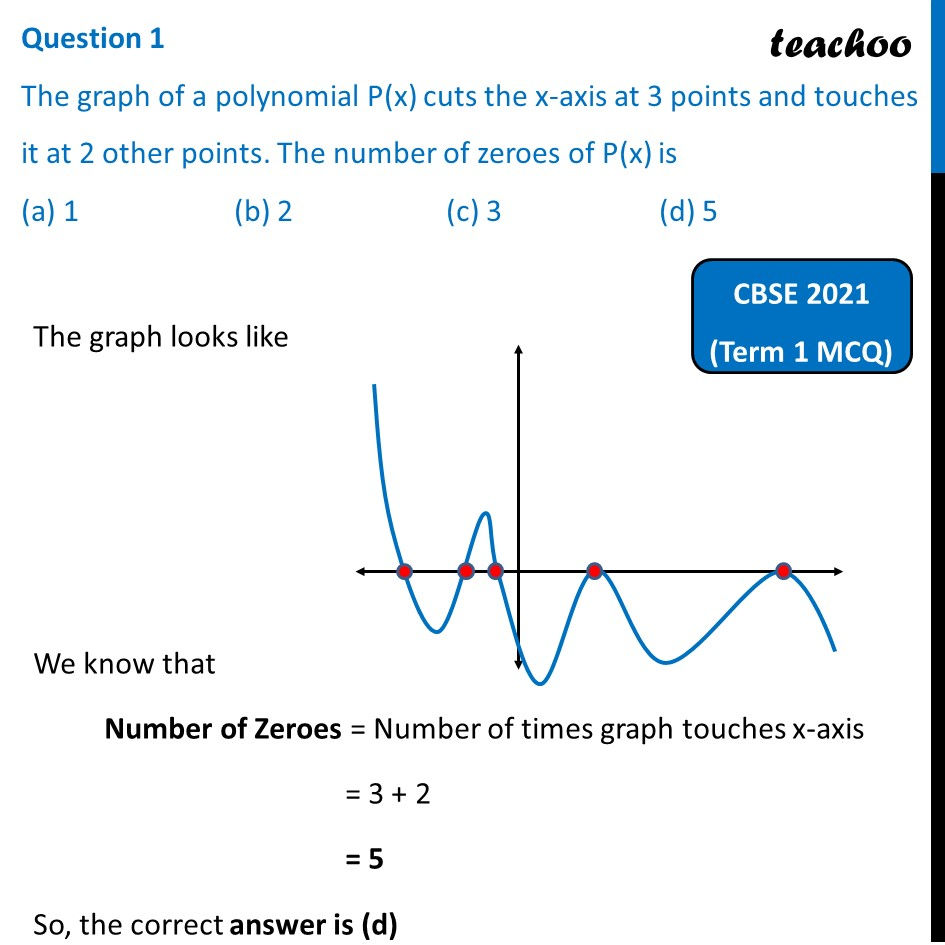Past Year MCQ (Maths Standard)

Chapter 2 Class 10 Polynomials
Serial order wise

## (d) 5

This question is similar from Ex 2.1, 1 - Chapter 2 Class 10 - PolynomialsLearn in your speed, with individual attention - Teachoo Maths 1-on-1 Class

### Transcript

Question 1 The graph of a polynomial P(x) cuts the x-axis at 3 points and touches it at 2 other points. The number of zeroes of P(x) is (a) 1 (b) 2 (c) 3 (d) 5 The graph looks like We know that Number of Zeroes = Number of times graph touches x-axis = 3 + 2 = 5 So, the correct answer is (d)